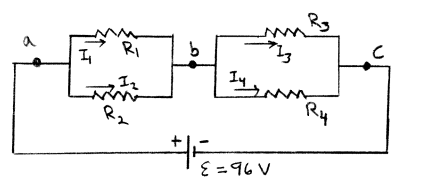# Problem: Consider the circuit shown in the sketch. R1 = 3.0 Ω, R2 = 6.0 Ω, R3 = 6.0 Ω. and R4 = 12.0 Ω. What is the potential difference Vab between points a and b and what is the potential difference Vbc between points b and c? a) Vab = b) Vbc =

90% (51 ratings)
###### Problem Details

Consider the circuit shown in the sketch. R1 = 3.0 Ω, R2 = 6.0 Ω, R3 = 6.0 Ω. and R4 = 12.0 Ω. What is the potential difference Vab between points a and b and what is the potential difference Vbc between points b and c?

a) Vab =

b) Vbc =